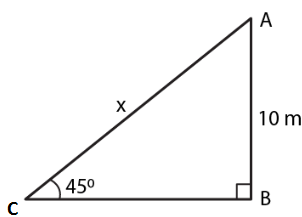Guru

# An electric pole is 10 m high. A steel wire tied to the top of the pole is affixed at a point on the ground to keep the pole upright. If the wire makes an angle of 450 with the horizontal through the foot of the pole, find the length of the wire.

• 0

sir this is the question from the book -ML aggarwal( avichal publication) class 10th , chapter20 , heights and distances
it is an important question , since it has been asked in exams.
we have been given that An electric pole is 10 m high.
A steel wire tied to the top of the pole is affixed at a point on the ground to keep the pole upright.
If the wire makes an angle of 450 with the horizontal through the foot of the pole,
find the length of the wire.
question no 9 , heights and distances , ICSE board, ML aggarwal

Share

1. Consider AB as the pole and AC as the wire which makes an angle of 450 with the ground.

Height of the pole AB = 10 m

Consider x m as the length of wire ACWe know that

sin θ = AB/AC

Substituting the values

sin 450 = 10/x

So we get

1/√2 = 10/x

By cross multiplication

x = 10√2 = 10 (1.414)

x = 14.14

Hence, the length of the wire is 14.14 m.

• 0